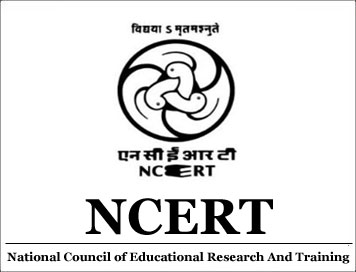NCERT Solutions for Class 4 Maths (Math Magic) Chapter 3 A Trip to Bhopal

Get here NCERT Solution for Class 4 Maths Chapter 3 for Free. NCERT Solutions for Maths Class 4 provided here are best answers by AglaSem School experts, who have many year of experience in this field. Class 4 NCERT Textbook Solutions cover all the exercises of each chapter. NCERT Solutions for Class 4 Maths Math Magic Book are given below.
Book Name: Math Magic
Subject: Maths
Chapter Number: 3
Chapter Name: A Trip to Bhopal
You can also bookmark this page by clicking + Save to Library icon and refer to these NCERT Solutions anytime from your account.

NCERT Solutions for Class 4 Maths (Math Magic)Chapter 5 The Way The World Looks

Get here NCERT Solution for Class 4 Maths Chapter 5 for Free. NCERT Solutions for Maths Class 4 provided here are best answers by AglaSem School experts, who have many year of experience in this field. Class 4 NCERT Textbook Solutions cover all the exercises of each chapter. NCERT Solutions for Class 4 Maths Math Magic Book are given below.
Book Name: Math Magic
Subject: Maths
Chapter Number: 5
Chapter Name: The Way The World Looks
You can also bookmark this page by clicking + Save to Library icon and refer to these NCERT Solutions anytime from your account.

NCERT Solutions for Class 4 Maths (Math Magic) Chapter 1 Building with Bricks

Get here NCERT Solution for Class 4 Maths Chapter 1 for Free. NCERT Solutions for Maths Class 4 provided here are best answers by AglaSem School experts, who have many year of experience in this field. Class 4 NCERT Textbook Solutions cover all the exercises of each chapter. NCERT Solutions for Class 4 Maths Math Magic Book are given below.
Book Name: Math Magic
Subject: Maths
Chapter Number: 1
Chapter Name: Building with Bricks
You can also bookmark this page by clicking + Save to Library icon and refer to these NCERT Solutions anytime from your account.

NCERT Solutions for Class 4 Maths (Math Magic) Chapter 4 Tick-Tick-Tick

Get here NCERT Solution for Class 4 Maths Chapter 4 for Free. NCERT Solutions for Maths Class 4 provided here are best answers by AglaSem School experts, who have many year of experience in this field. Class 4 NCERT Textbook Solutions cover all the exercises of each chapter. NCERT Solutions for Class 4 Maths Math Magic Book are given below.
Book Name: Math Magic
Subject: Maths
Chapter Number: 4
Chapter Name: Tick-Tick-Tick
You can also bookmark this page by clicking + Save to Library icon and refer to these NCERT Solutions anytime from your account.

NCERT Solutions for Class 4 Maths (Math Magic) Chapter 6 The Junk Seller

Get here NCERT Solution for Class 4 Maths Chapter 6 for Free. NCERT Solutions for Maths Class 4 provided here are best answers by AglaSem School experts, who have many year of experience in this field. Class 4 NCERT Textbook Solutions cover all the exercises of each chapter. NCERT Solutions for Class 4 Maths Math Magic Book are given below.
Book Name: Math Magic
Subject: Maths
Chapter Number: 6
Chapter Name: The Junk Seller
You can also bookmark this page by clicking + Save to Library icon and refer to these NCERT Solutions anytime from your account.